ishritam/Quora-question-pair-similarity

Quora has given an (almost) real-world dataset of question pairs, with the label of is_duplicate along with every question pair. The objective was to minimize the logloss of predictions on duplicacy in the testing dataset. Given a pair of questions q1 and q2, train a model that learns the function: f(q1, q2) → 0 or 1 where 1 represents that q1 a…
Jupyter NotebookFetching latest commit…
Cannot retrieve the latest commit at this time.
Type Name Latest commit message Commit time
Failed to load latest commit information.1.Quora.ipynb2.Quora_Preprocessing.ipynb3. TF_IDF vectors.ipynbQuora Case Study.ipynbREADME.md

Quora-question-pair-similarity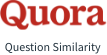Quora is the place where questions get answers. This website helps online users raise queries for knowing the unknown and reply to ones they are good at answering. There are 7.6 billion people living on the planet and over 100 million people visit Quora every month, so it's no surprise that many people ask similarly worded questions. Multiple questions with the same intent can cause seekers to spend more time finding the best answer to their question and make writers feel they need to answer multiple versions of the same question. Quora values canonical questions because they provide a better experience to active seekers and writers, and offer more value to both of these groups in the long term.Quora has given an (almost) real-world dataset of question pairs, with the label of is_duplicate along with every question pair. The objective was to minimize the logloss of predictions on duplicacy in the testing dataset. Given a pair of questions q1 and q2, train a model that learns the function:
f(q1, q2) → 0 or 1
where 1 represents that q1 and q2 have the same intent and 0 otherwise.

Problem Statement

• Identify which questions asked on Quora are duplicates of questions that have already been asked.
• This could be useful to instantly provide answers to questions that have already been answered.
• We are tasked with predicting whether a pair of questions are duplicates or not.

Real world/Business Objectives and Constraints

1. The cost of a mis-classification can be very high.
2. You would want a probability of a pair of questions to be duplicates so that you can choose any threshold of choice.
3. No strict latency concerns.
4. Interpretability is partially important.

Data Overview

- Data will be in a file Train.csv
- Train.csv contains 5 columns : qid1, qid2, question1, question2, is_duplicate
- Size of Train.csv - 60MB
- Number of rows in Train.csv = 404,290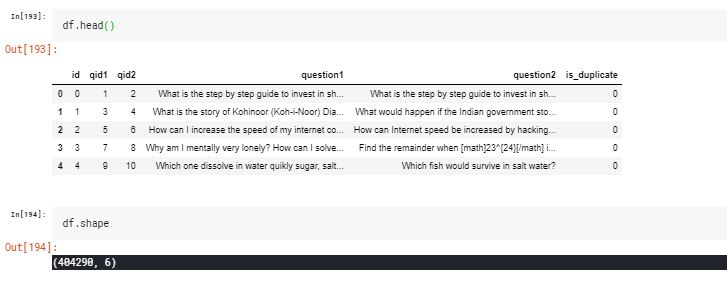Performance Metric

• log-loss
• Binary Confusion Matrix

Some Data Analysis

• Distribution of data points among output classes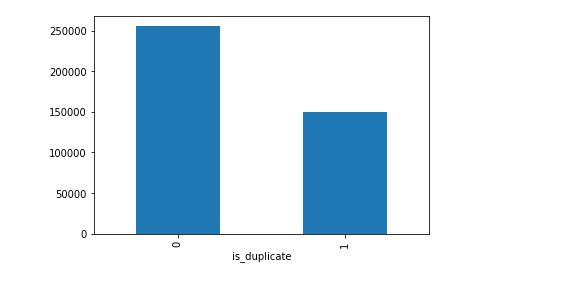• Number of unique questions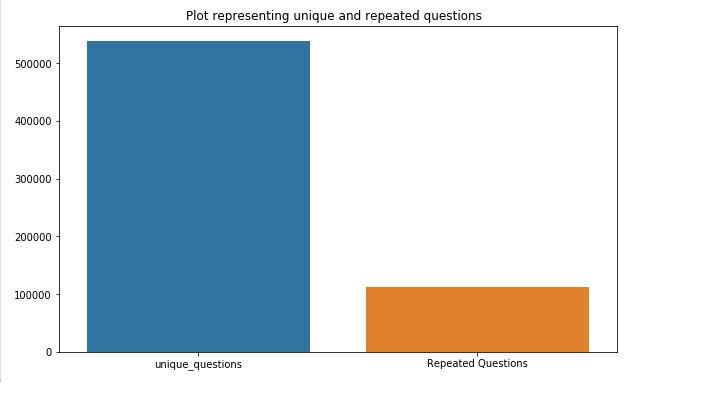• Wordcloud for similar questions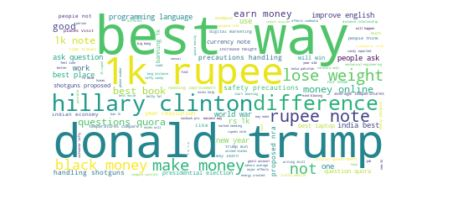• Wordcloud for dissimilar questions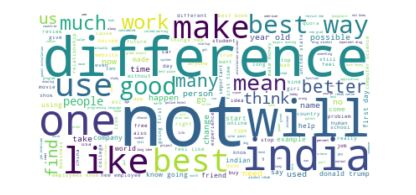As it's a high-level dataset, entry-level machines with 8GB RAM will take so much of time for the executions. That's why I switched to Kaggle Kernel for this case study. First I load some basic requirement packages for this case study.

Creating a SQLite database

I created an SQLite database and wrote records stored in a DataFrame df to a SQL database. And Considered less number of data point randomly like ~100k.

Preprocessing

1. Deduplication of entries
2. Checking for NULL values
3. Preprocessing of Text:
- Removing html tags
- Removing Punctuations
- Performing stemming
- Removing Stopwords
- Expanding contractions etc.

4. Removing  empty question rows if any exist

Feature Extraction

Basic Feature Extraction

• freq_qid1 = Frequency of qid1's
• freq_qid2 = Frequency of qid2's
• q1len = Length of q1
• q2len = Length of q2
• q1_n_words = Number of words in Question 1
• q2_n_words = Number of words in Question 2
• word_Common = (Number of common unique words in Question 1 and Question 2)
• word_Total =(Total num of words in Question 1 + Total num of words in Question 2)
• word_share = (word_common)/(word_Total)
• freq_q1+freq_q2 = sum total of frequency of qid1 and qid2
• freq_q1-freq_q2 = absolute difference of frequency of qid1 and qid2

Definition:

• Token: You get a token by splitting sentence a space
• Stop_Word : stop words as per NLTK.
• Word : A token that is not a stop_word

Features:

• cwc_min : Ratio of common_word_count to min lenghth of word count of Q1 and Q2
cwc_min = common_word_count / (min(len(q1_words), len(q2_words))

- __cwc_max__ : Ratio of common_word_count to max lenghth of word count of Q1 and Q2
cwc_max = common_word_count / (max(len(q1_words), len(q2_words))

- __csc_min__ : Ratio of common_stop_count to min lenghth of stop count of Q1 and Q2
csc_min = common_stop_count / (min(len(q1_stops), len(q2_stops))

- __csc_max__ : Ratio of common_stop_count to max lenghth of stop count of Q1 and Q2
csc_max = common_stop_count / (max(len(q1_stops), len(q2_stops))

- __ctc_min__ : Ratio of common_token_count to min lenghth of token count of Q1 and Q2
ctc_min = common_token_count / (min(len(q1_tokens), len(q2_tokens))

• ctc_max : Ratio of common_token_count to max lenghth of token count of Q1 and Q2
ctc_max = common_token_count / (max(len(q1_tokens), len(q2_tokens))

• last_word_eq : Check if First word of both questions is equal or not
last_word_eq = int(q1_tokens[-1] == q2_tokens[-1])

• first_word_eq : Check if First word of both questions is equal or not
first_word_eq = int(q1_tokens == q2_tokens)

• abs_len_diff : Abs. length difference
abs_len_diff = abs(len(q1_tokens) - len(q2_tokens))

• mean_len : Average Token Length of both Questions
mean_len = (len(q1_tokens) + len(q2_tokens))/2

• longest_substr_ratio : Ratio of length longest common substring to min length of the token count of Q1 and Q2 longest_substr_ratio = len(longest common substring) / (min(len(q1_tokens), len(q2_tokens)) Some Features analysis and visualizations word_share - We can check from below that it is overlapping a bit, but it is giving some classifiable score for dissimilar questions.

Some Features analysis and visualizations

• word_share - We can check from below that it is overlaping a bit, but it is giving some classifiable score for disimilar questions.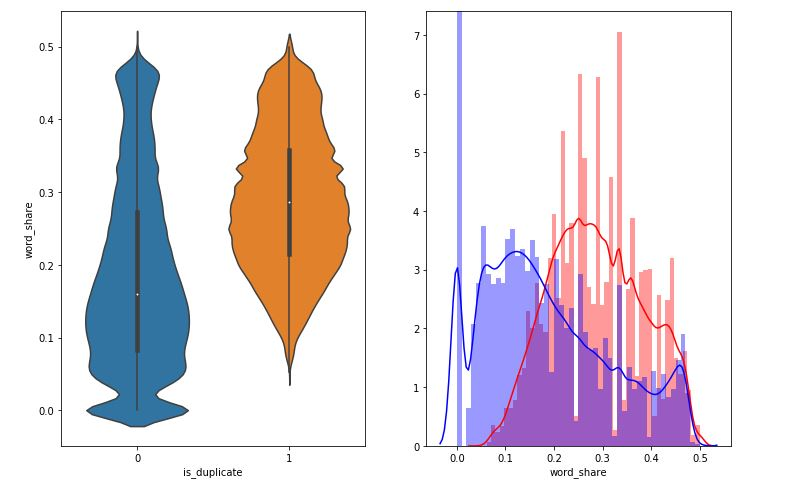• Word Common - it is almost overlaping.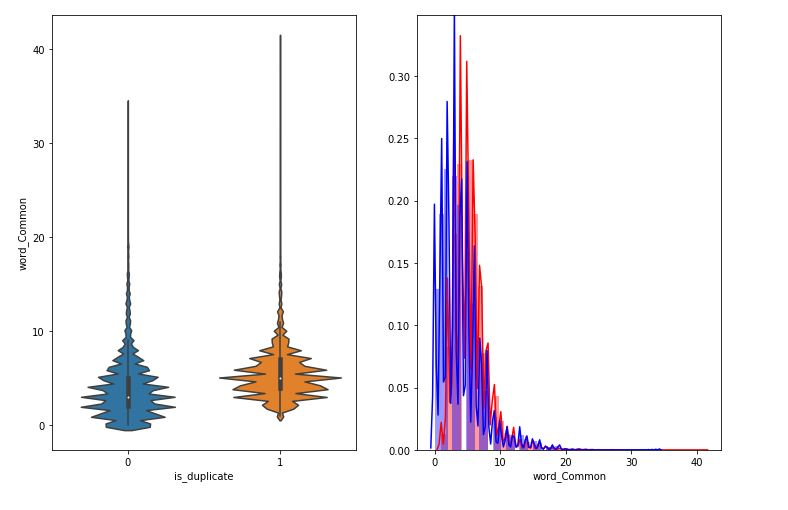• Pair plot of features ['ctc_min', 'cwc_min', 'csc_min', 'token_sort_ratio']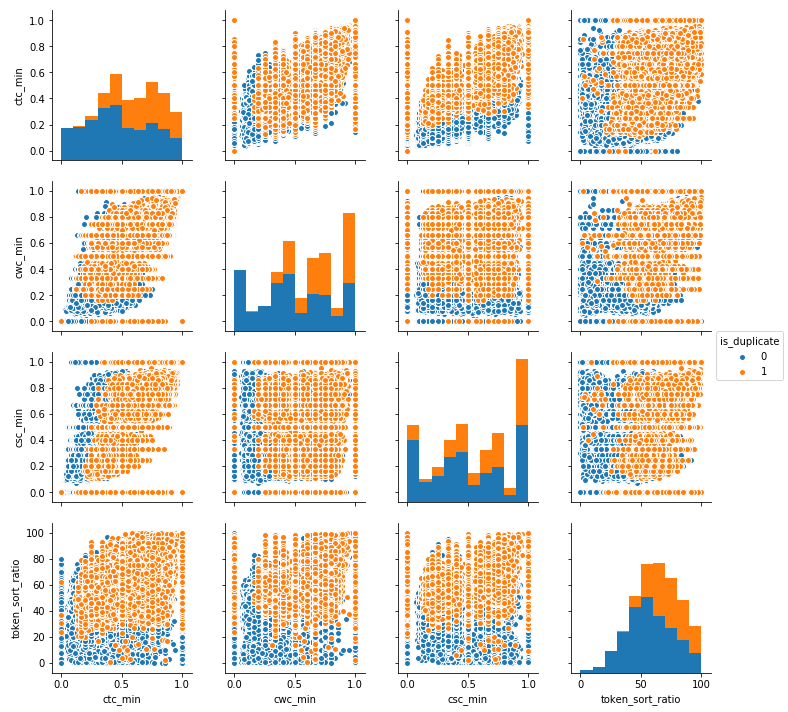Machine Learning Models

• Building a random model (Finding worst-case log-loss)

Using a random model to get the upper bound for the log-loss. I want the value of log-loss to be lesser than the log-loss of a random model. Trained a random model to check Worst case log loss and got log loss as ** 0.887019447220453 **.

• Random train test split( 70:30)

Splited the data in to 70 30 ratio

• Vectorization

Vectorized the data with simple TF-IDF vectors.

• Models

Trained LR, SVM models and also tuned hyperparameters using Random Search. Hyperparameter tuned XgBoost using RandomSearch to reduce the log-loss. Below are models and their log loss scores.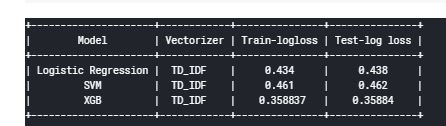• Featurized text data with tfidf weighted word-vectors
• Models

Trained LR, SVM models with tfidf weighted word-vectors and also tuned hyperparameters using Random Search. Hyperparameter tuned XgBoost using RandomSearch to reduce the log-loss. Below are models and their log loss scores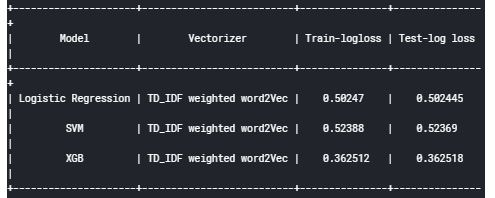Reference:

1. Kaggle Discussion https://www.kaggle.com/anokas/data-analysis-xgboost-starter-0-35460-lb/comments
2. Kaggle Winning Solution and other approaches: https://www.dropbox.com/sh/93968nfnrzh8bp5/AACZdtsApc1QSTQc7X0H3QZ5a?dl=0
3. Blog 1 : https://engineering.quora.com/Semantic-Question-Matching-with-Deep-Learning
4. Blog 2 : https://towardsdatascience.com/identifying-duplicate-questions-on-quora-top-12-on-kaggle-4c1cf93f1c30
You can’t perform that action at this time.